# Adaptive Energy Efficient Transmission in WSNs

2755 words (11 pages) Essay in Computer Science

26/07/18 Computer Science Reference this

Disclaimer: This work has been submitted by a student. This is not an example of the work produced by our Essay Writing Service. You can view samples of our professional work here.

Any opinions, findings, conclusions or recommendations expressed in this material are those of the authors and do not necessarily reflect the views of UK Essays.

### A novel approach for adaptive energy efficient transmission in WSNs

• S Murthy Vedireswarapu, Muni Bhaskar. Athikayala Shankar.T

### Abstract

In wireless sensor networks (WSNs), sensor nodes work on finite capacity batteries. So, in order to increase network lifetime the mobile sensor nodes should be operated at low power. Hence, we need a novel approach for efficient scheme for transmission in WSNs. In this scheme, according to temperature changes the connectivity between sensor nodes is estimated by using open loop process, the network is divided into three regions based on closed loop feedback process which helps to reduce packets overhead in the network. Region dependent threshold on loss of transmitter power (RSloss) and present number of nodes in each region helps to adjust transmission power level according to changes in connectivity between nodes because of changes in temperature. This scheme achieves high energy saving than existing conventional method.

Index Termsthreshold on transmitter power loss, wireless sensor networks (WSNs), transmitter power level.

## I. INTRODUCTION

Wireless sensor networks (WSNs) works based on IEEE 802.15.4 standard. This standard operates at frequency of 2.4GHZ and data rate of 250 kbps. WSNs are used mainly in industrial, bio medical, security surveillance and weather monitoring applications. Each sensor node consists of: radio transmitter, receiver, sensing unit, processing unit and limited capacity battery  .Because of limited battery lifetime at each sensor node, the transmitter power level should be minimized to increase the energy efficiency and network lifetime of WSNs. In WSNs all the systems should be operate at low power to increase energy efficiency because it is an important concern in wireless communication.

To collect data from different environments sensor nodes are deployed at different locations within WSNs. While the communication between sensor nodes they consume less power as sensor nodes operate on limited battery, and temperature effects the connectivity between the sensor nodes. So, we need to reduce energy consumption for communication between sensor nodes, and at the same time ensure that good connectivity between sensor nodes in order to increase network life time [2-4].we analyzed a new scheme for Ptlevel to efficiently adjust the connectivity between nodes due to temperature changes. This scheme improves network lifetime while maintaining good connectivity between sensor nodes. By using temperature sensor, the connectivity between each node is estimated in open loop process. Estimated degradation in connectivity is compensated by using closed loop feedback process in the new scheme. In this scheme we obtain less transmission power compared to existing schemes.

## II. LITERATURE REVIEW

In algorithm of local mean (LMA), Based on number of received acknowledgements by the reference node the transmission Plevel is adjusted according to threshold transmission Ptlevel .It improves network lifetime but cannot estimate the link quality . In other existing schemes based on RSloss, transmitter Ptlevel is estimated .

In other existing scheme closed loop feedback process is used for connectivity estimation .In this scheme each node aware of required Ptlevel to be maintained with its adjacent nodes. According to connectivity changes it adjusts the transmission power level .The above schemes cannot guarantee both low power transmission and good connectivity between nodes. The new scheme efficiently adjusts the connectivity changes according to temperature changes. Based on RSloss ,Ptlevel is estimated for all three regions for good connectivity between the nodes.

## III. PROBLEM STATEMENT

In WSNs due to multipath propagation signal strength varies irregularly, the signal strength mainly depends on the transmitted power at any time. The reason for the variation in the RSloss .First the variation in signal strength according to distance due to multipath effect, secondly the environmental factors like temperature effects the signal strength. So the temperature is one of the main factors which affect the RSloss and transmission power level. Hence we need to compensate these parameters according to surrounding temperature variations.

## IV. SOLVING METHODOLOGY

In this scheme open loop feedback process uses temperature sensor to detect the temperature variations. In closed loop feedback process, transmission Ptlevel is adjusted based on control packets overhead. Based on open loop and closed loop feedback process the network is divided into three logical regions: as high, medium and low represented with X, Y and Z respectively. In this scheme we use RSloss to measure the connectivity with relatively low overhead.

Open loopnc(t)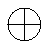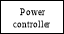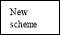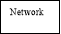nd(t)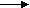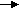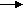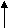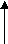Closed loop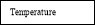Figure 1: Block diagram

## V. ENERGY-EFFICIENT TRANSMISSION SCHEME.

In this scheme, transmission Ptlevel is adjusted by the power controller by utilizing the current number of nodes and temperature sensed by each node. Temperature compensation is done in case of any changes in temperature sensed at a sensor by using the relation between temperature and RSloss. Transmission power loss due to temperature variation is given as 

RSloss[dBm]=0.1996*(T[0C]-25[0C]).

By using LSA the transmitter Ptlevel is obtained as follows 

Ptlevel=[(RSloss+40)/12]^2.91

According to free space model the actual transmitter power level is obtained as follows,

Ptr[dBm]=[ Æž*( Eb/No)*mkTB*(4Πd/λ)2+RNF]+RSloss

Here number of nodes (N), distance between each node (d),signal to noise ratio(Eb/No), spectral efficiency(Æž), frequency(f) and Receiver noise figure(RNF). 

The main variables are transmitter power loss and transmitter power in each region decides the working of working of algorithm.

A beacon message is broadcasted periodically by the reference node and waits for ACKs. If ACKs are received from nodes, RSloss is estimated for division of network logically, considering region X with high RSloss, region Y with medium RSloss and low RSloss as Z region. Estimating of transmitter Ptlevel is as follows: If RSloss < RSloss threshold then default transmitter Ptlevel is assigned. In other case if RSloss ≥ RSloss threshold and nc(t) ≥ nd(t) ,then threshold Ptlevel is assigned. For similar case with nc(t) < nd(t) transmitter Ptlevel is assigned.

Minimum, Maximum and Average value of RSloss for all nodes present in the network can formulated as follows:

RSloss(min)=min(RSloss(k)),

RSloss(max)=max(RSloss(k)),

RSloss (Avg) = (min(RSloss(k)) + max(RSloss(k)))/2

Then limits of the three regions are given as: 

For region X:

RSloss( X max)=max(RSloss(k)),

RSloss( X min)= (RSloss(Avg)) + 2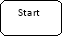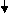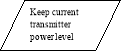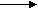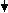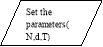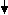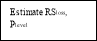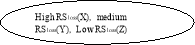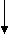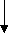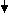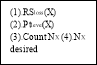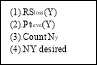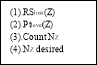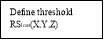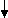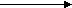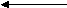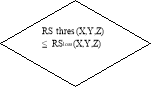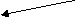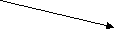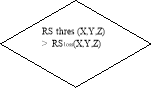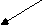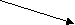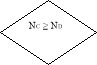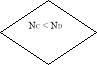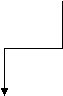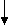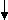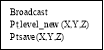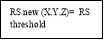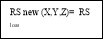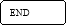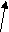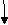Figure 2: Flow chart of reference node 

To count number of nodes in each region, a counter is initialized with initially zero.

For region Y:

RSloss( Y max)=(RSloss(Avg)) + 2

RSloss( Y min)=(RSloss(Avg)) – 2

For region Z:

RSloss( Z max)=(RSloss(Avg)) – 2

RSloss( Z min)=min(RSloss(k)) ; k ε N

RSloss( X Threshold) =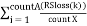RSloss( Y Threshold) =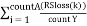RSloss( Z Threshold) =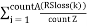RSloss­-new(X,Y,Z)(k)=RSloss(Threshold X,Y,Z)

Given that for all k É› N,

RSloss(Threshold X,Y,Z)≤ RSloss(X,Y,Z)(k)

And nc(t)(X,Y,Z) ≥ nd(t)(X,Y,Z)

RSloss-new(X,Y,Z)(k)=RSloss(X,Y,Z)(k)

Given that for all k ε N,

RSloss(Threshold X,Y,Z) ≤ RSloss (X,Y,Z)(k)

And nc(t)(X,Y,Z) ≤ nd(t)(X,Y,Z) or

RSloss(Threshold X,Y,Z)>RSloss(X,Y,Z)(k)

Estimation of Ptlevel for new RSloss is formulated as for all k É› N,

Ptlevel-new(X,Y,Z)(k)= [(RSloss-new(X,Y,Z)(k)+40)/12]^2.91

The difference between Ptlevels assigned before and after the proposed scheme is denoted as Ptsave.

Ptsave (X,Y,Z) =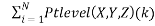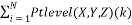## VI. SIMULATION RESULTS

 Simulation Parameters Values Rounds 10 Temperature 10º-53ºC Distance (1-100)m Nodes 100 Regions X,Y,Z Æž 0.0029 Bandwidth 83.5 MHz Frequency 2.45 GHz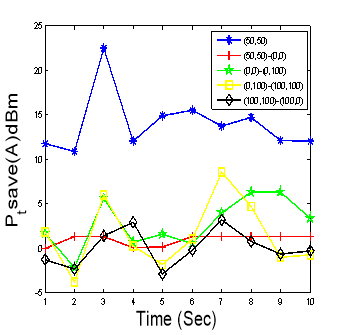Figure 3: Transmitter power saved in region X for different reference node location.

From figure 3, we can infer that, maximum Ptsave is 12dBm to 21dBm. When a reference node is at origin of the square region, Ptsave is constant around 1dBm.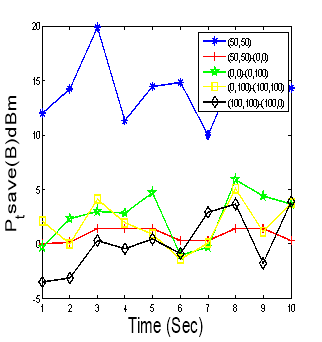Figure 4: Transmitter Ptsave in region Y for different reference node location. From above figure, we can infer that maximum. Ptsave for region Y varies from 12dBm to 21dBm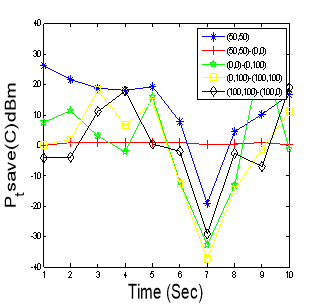Figure 5: Transmitter Ptsave in region Z for different reference location.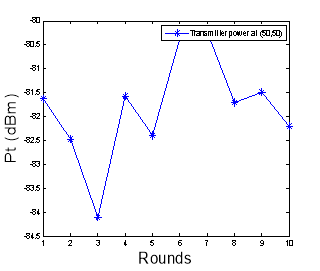Figure 6: Transmitter power for different rounds.

From above figure 5, we can infer that Ptsave for region Z varies from -20dBm to 20dBm.

From figure 6, we can clearly observe that Pt lies between -84dBm to – 80dBm.

## VII. CONCLUSION

By using this scheme the energy consumption of the mobile nodes is reduced in WSNs. This scheme uses both open loop control and closed loop feedback control process. The temperature changes are adjusted with the help of open loop process. By using these two processes the energy consumption of mobile sensor nodes is reduced by comparing with existing schemes. By dividing the network into three different regions we can prolong the lifetime of network and maintains good connectivity between sensor nodes.

## Related Services

View all

### DMCA / Removal Request

If you are the original writer of this essay and no longer wish to have the essay published on the UK Essays website then please:Prices from
###### £28

Undergraduate 2:2 • 250 words • 7 day delivery

Delivered on-time or your money back

##### Rated 4.6 out of 5 by(135 Reviews)